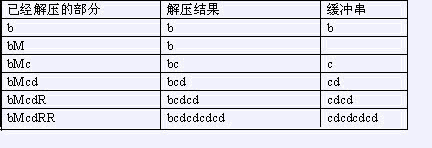# bzoj1068 [SCOI2007]压缩（区间DP）

## bzoj1068 [SCOI2007]压缩

给一个由小写字母组成的字符串，我们可以用一种简单的方法来压缩其中的重复信息。压缩后的字符串除了小写字母外还可以（但不必）包含大写字母R与M，其中M标记重复串的开始，R重复从上一个M（如果当前位置左边没有M，则从串的开始算起）开始的解压结果（称为缓冲串）。
bcdcdcdcd可以压缩为bMcdRR，下面是解压缩的过程：1<=n<=50

dp[l][r][0/1]表示在默认前边(l-1)有一个M的情况下，

dp[l][r]=min(dp[l][k]+rk)$dp[l][r]=min(dp[l][k]+r-k)$（因为dp[][][]默认前边有一个M，所以不是加dp[k+1][r]）
dp[l][r]=dp[l][mid]+1$dp[l][r]=dp[l][mid]+1$ 仅当l-r+1位偶数且两半相同时，在后边添一个R。

dp[l][r]=min( min(dp[l][k],f[l][k])+1+min(dp[k+1][r],dp[k+1][r]) )$dp[l][r]=min( \ min(dp[l][k],f[l][k])+1+min(dp[k+1][r],dp[k+1][r])\ )$

#include<cstdio>
#include<iostream>
#include<cstring>
#include<algorithm>
using namespace std;
const int N=55;
const int inf=0x3f3f3f3f;
int f[N][N],n;
char s[N];
bool check(int l,int mid,int r)
{
for(int i=l;i<=mid;i++)
if(s[i]!=s[mid+i-l+1]) return 0;
return 1;
}
int dp(int l,int r,int m)
{
int len=(r-l+1);
if(len==1) return f[l][r][m]= m?inf:1;
if(f[l][r][m]<inf) return f[l][r][m];
if(m)
{
for(int k=l;k<r;k++)
f[l][r][m]=min(f[l][r][m],min(dp(l,k,0),dp(l,k,1))+min(dp(k+1,r,0),dp(k+1,r,1))+1);
return f[l][r][m];
}
int mid=(l+r)>>1;
if(len%2==0&&check(l,mid,r)) f[l][r][m]=min(f[l][r][m],dp(l,mid,m)+1);
for(int k=l;k<=r;k++) f[l][r][m]=min(f[l][r][m],dp(l,k,m)+r-k);
return f[l][r][m];
}
int main()
{
scanf("%s",s+1); n=strlen(s+1);
memset(f,0x3f,sizeof(f));
printf("%d\n",min(dp(1,n,0),dp(1,n,1)));
return 0;
}

©️2019 CSDN 皮肤主题: 深蓝海洋 设计师: CSDN官方博客